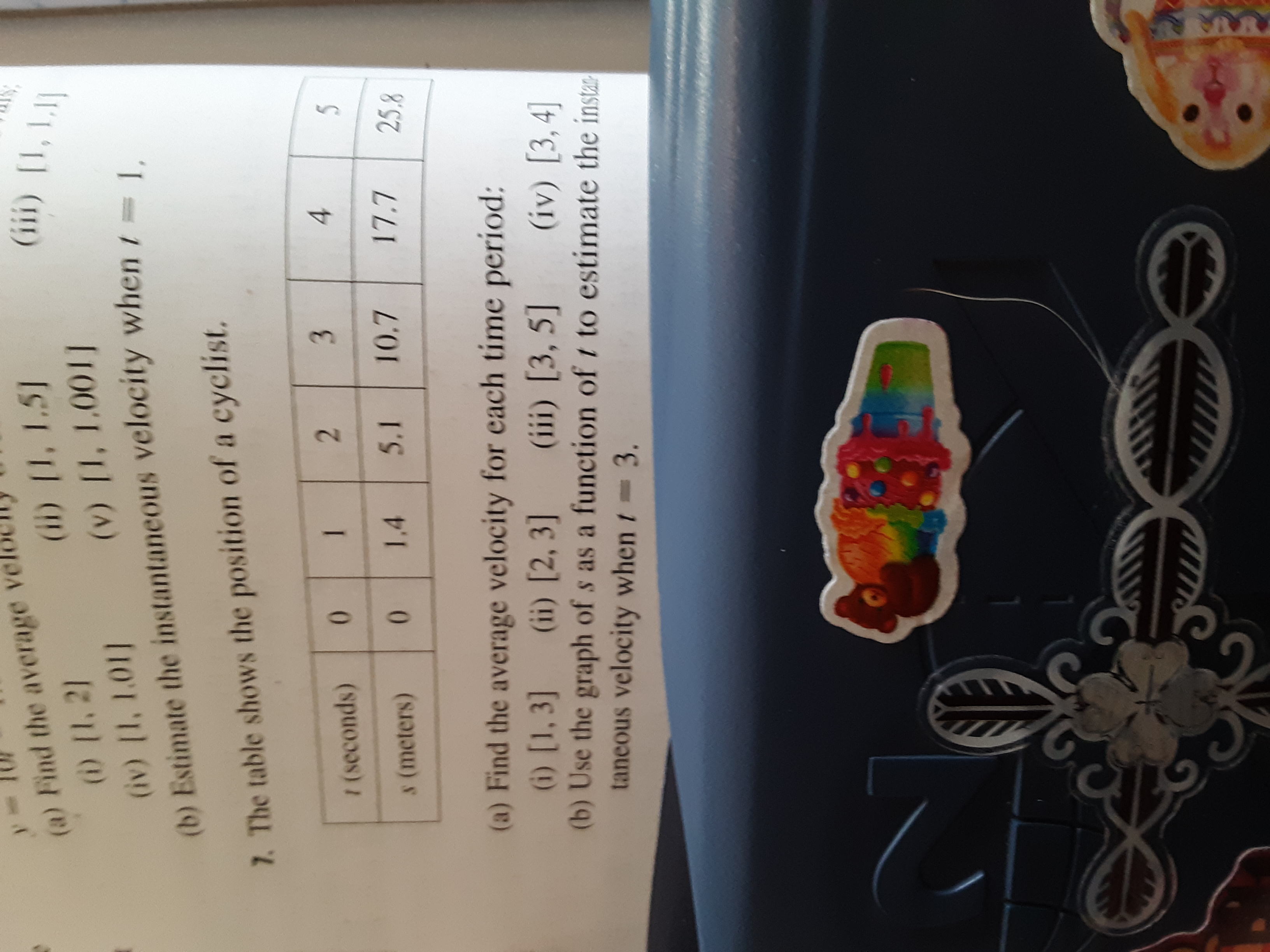# (a) Find the average(i) [1, 21(iv) [1, 1.01](b) Estimate the instantaneous velocity when t=1,(iii) I,L1(ii) [I, 1.51(v) [1, 1.001]7. The table shows the position of a cyclist.3.1/ (seconds)510.717.75.11.4s (meters)25.8(a) Find the average velocity for each time period:(i) [1, 3](b) Use the graph of s as a function of t to estimate the instataneous velocity when t 3.(ii) [2, 3](iii) [3, 5](iv) [3, 4]

Question
116 views

Is there a formula for finding instantaneous velocity, and if not, can you show me how to find it using a set of given data? (Pic below)help_outlineImage Transcriptionclose(a) Find the average (i) [1, 21 (iv) [1, 1.01] (b) Estimate the instantaneous velocity when t=1, (iii) I,L1 (ii) [I, 1.51 (v) [1, 1.001] 7. The table shows the position of a cyclist. 3. 1 / (seconds) 5 10.7 17.7 5.1 1.4 s (meters) 25.8 (a) Find the average velocity for each time period: (i) [1, 3] (b) Use the graph of s as a function of t to estimate the insta taneous velocity when t 3. (ii) [2, 3] (iii) [3, 5] (iv) [3, 4] fullscreen
check_circle

Step 1

(a) Find the average velocity for each time period:

Step 2
Step 3

(b) Estimate the instantaneo...

### Want to see the full answer?

See Solution

#### Want to see this answer and more?

Solutions are written by subject experts who are available 24/7. Questions are typically answered within 1 hour.*

See Solution
*Response times may vary by subject and question.
Tagged in

### Calculus